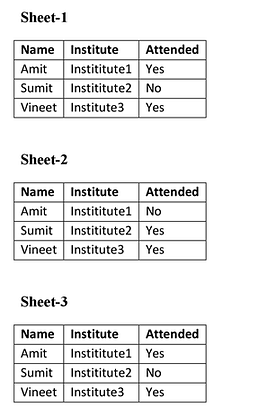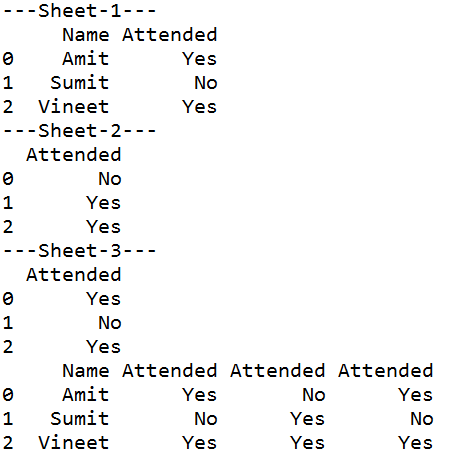## Saturday, June 20, 2020

### Import and Match Multiple DataFrames from EXCEL using PYTHON PANDAS

Many times, there is need to concatenate and analyze multiple data frames in Python.

Suppose we have Three different Excel Sheets in which same type of attributes are there. From these excel sheets, the data can be imported to data frames and then analysis can be done.

Following are three different MS Excel Sheets of attendance of the candidates. From these sheets, we have to check whether a candidate attended all sessions or not`PYTHON CODE`
`import pandas as pddf1 = pd.read_excel (r'Book1.xlsx')df1 = pd.DataFrame(df1, columns= ['Name', 'Attended'])df2 = pd.read_excel (r'Book2.xlsx')df2 = pd.DataFrame(df2, columns= ['Attended'])df3 = pd.read_excel (r'Book3.xlsx')df3 = pd.DataFrame(df3, columns= ['Attended'])print('---Sheet-1---')print (df1)print('---Sheet-2---')print (df2)print('---Sheet-3---')print (df3)frames = [df1, df2, df3]result = pd.concat(frames, axis=1, sort=False)print(result)`

Output